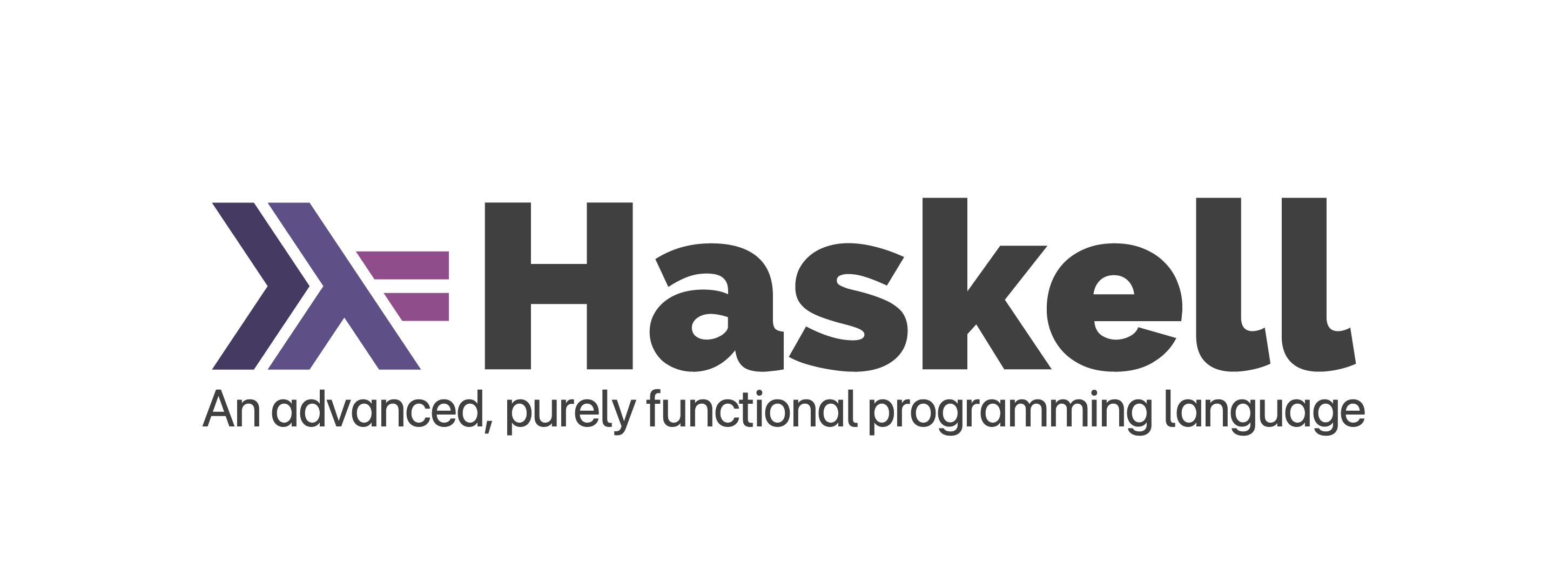Back## 为何需要类型？

TL;DR：lol，看看 JavaScript。

## 类型签名

ghci> :t 't'
't' :: Char
ghci> :t "Hello"
"Hello" :: String
ghci> :t False
False :: Bool

ghci> :t a
a :: Num p => p
ghci> b = 13 :: Integer
ghci> :t b
b :: Integer

## 函数类型

ghci> :i (->)
data (->) a b
ghci> :i (,)
data (,) a b = (,) a b

fst :: (a, b) ->   a
--          
1. 第一个形参为 (a, b) 类型，一个双元组。
2. 函数类型，(->)，有两个形参，一个是 (a, b) 另一个是结果 a
3. 函数的结果类型 a，和元组中的 a 一致。
length :: [a] -> Int

length 函数有一个形参 [a]，是一个列表，并且返回 Int 结果。该函数不关心列表的元素类型，因此没有类型限制。

### 类型限制

(+) :: Num a => a -> a -> a
(/) :: Fractional a => a -> a -> a

ghci> fifteen = 15
ghci> :t fifteen
fifteen :: Num p => p
ghci> fifteenInt = fifteen :: Int
ghci> fifteenDouble = fifteen :: Double
ghci> :t fifteenInt
fifteenInt :: Int
ghci> :t fifteenDouble
fifteenDouble :: Double
ghci> :info Num
...
instance Num Int -- Defined in ‘GHC.Num’
instance Num Double -- Defined in ‘GHC.Float’

NumIntDouble 相加是可行的：

ghci> fifteen + fifteenDouble
30.0
ghci> fifteen + fifteenInt
30

ghci> fifteenDouble + fifteenInt
<interactive>:40:18: error:
• Couldn't match expected type ‘Double’ with actual type ‘Int’
• In the second argument of ‘(+)’, namely ‘fifteenInt’
In the expression: fifteenDouble + fifteenInt
In an equation for ‘it’: it = fifteenDouble + fifteenInt

(Num a, Num b) => a -> b -> b
-- or
(Ord a, Num a) => a -> a -> Ordering

## 柯里化

(+) :: Num a => a -> a -> a
|    1   |    2    | 
1. 类型限制，要求 a 必须具有 Num 实例。
2. 通过将函数嵌套，可以实现类似多个参数的效果。连续应用函数，每次都接受一个参数并返回值。
3. 返回类型为 a 的值

f :: a -> a -> a
f :: a -> (a -> a)
map :: (a -> b) -> [a] -> [b]
map :: (a -> b) -> ([a] -> [b])

### 部分应用

addStuff :: Integer -> Integer -> Integer
addStuff a b = a + b + 5
ghci> :t addStuff
addStuff :: Integer -> Integer -> Integer
addStuff :: Integer -> Integer -> Integer
ghci> fifteen
15
25
15

### 手动柯里和反柯里

Haskell 默认柯里化，但也可以反柯里化函数。反柯里化（uncurrying）意味着将嵌套结构铺平，并用相应个数的元组替代。如果反柯里化 (+)，类型签名将从 Num a => a -> a -> a 变为 Num a => (a, a) -> a

• 反柯里化的函数：一个函数多个实参
• 柯里化的函数：很多函数，每次单个实参
nonsense :: Bool -> Integer
nonsense True = 805
nonsense False = 31337

curriedFunction :: Integer -> Bool -> Integer
curriedFunction i b =
i + nonsense b

uncurriedFunction :: (Integer, Bool) -> Integer
uncurriedFunction (i, b) = i + nonsense b

anonymous :: Integer -> Bool -> Integer
anonymous = \i b -> i + nonsense b

anonNested :: Integer -> Bool -> Integer
anonNested = \i -> \b -> i + nonsense b
ghci> curriedFunction 10 False
31347
ghci> anonymous 10 False
31347
ghci> anonNested 10 False
31347

### 柯里化和反柯里化现存函数

ghci> curry f a b = f (a, b)
ghci> :t curry
curry :: ((a, b) -> t) -> a -> b -> t
ghci> :t fst
fst :: (a, b) -> a
ghci> :t curry fst
curry fst :: t -> b -> t
ghci> fst (1, 2)
1
ghci> curry fst 1 2
1

ghci> uncurry f (a, b) = f a b
ghci> :t uncurry
uncurry :: (t1 -> t2 -> t3) -> (t1, t2) -> t3
ghci> :t (+)
(+) :: Num a => a -> a -> a
ghci> (+) 1 2
3
ghci> uncurry (+) (1, 2)
3

### 分片

ghci> let x = 5
ghci> let y = (2^)
ghci> let z = (^2)
ghci> y x
32
ghci> z x
25

ghci> let celebrate = (++ " woot!")
ghci> celebrate "naptime"
"naptime woot!"
ghci> celebrate "dogs"
"dogs woot!"

ghci> elem 9 [1..10]
True
ghci> 9 elem [1..10]
True
ghci> let c = (elem [1..10])
ghci> c 9
True
ghci> c 25
False

ghci> let hasTen = elem 10
ghci> hasTen [1..9]
False
ghci> hasTen [5..15]
True

## 多态性

id :: a -> a

Prelude> id 1
1
Prelude> id "blah"
"blah"
Prelude> id [(1,2,3), (1,2,3), (2,3,4)]
[(1,2,3),(1,2,3),(2,3,4)]

Num a => a -> a

### 多态常量

Haskell 具有类型限制，但直觉上而言，我们希望 -10 + 6.3 这种运算能够通过编译：

Prelude> (-10) + 6.3
-3.7

 Prelude> :t (-10) + 6.3
(-10) + 6.3 :: Fractional a => a
Prelude> :t (-10)
(-10) :: Num a => a

Prelude> let x = 5 + 5
Prelude> :t x
x :: Num a => a
Prelude> let x = 5 + 5 :: Int
Prelude> :t x
x :: Int

### 类型转换

Prelude> 6 / length [1, 2, 3]
No instance for (Fractional Int) arising
from a use of ‘/’

fromIntegral :: (Num b, Integral a) => a -> b
Prelude> 6 / fromIntegral (length [1, 2, 3])
2.0

## 类型推断

Prelude> myGreet x = "Hello, " ++ x
Prelude> :t myGreet
myGreet :: [Char] -> [Char]

Prelude> let myGreet x y = x ++ y
Prelude> :type myGreet
myGreet :: [a] -> [a] -> [a]

### 为声明断言类型

-- type declaration
triple :: Integer -> Integer
-- function declaration
triple x = x * 3

triple x = tripleItYo x
where tripleItYo :: Integer -> Integer
tripleItYo y = y * 3

Prelude> tripleItYo y = y * 3
Prelude> :t tripleItYo
tripleItYo :: Num a => a -> a

Prelude> let x = 5 + 5 :: String
No instance for (Num String) arising from a use of ‘+’
In the expression: 5 + 5 :: String
In an equation for ‘x’: x = 5 + 5 :: String